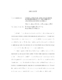## Computational and Analytical Investigations of Disordered and Interacting SystemsLocalization of particle wavefunctions in quasi-disordered one dimensional incommensurate lattices is studied both numerically and analytically. Through exact diagonalization, we show that energy dependent mobility edges can appear in the case of shallow lattices. We also show that these mobility edges can be studied with a tight-binding model (an extension of the Aubry-Andre model) that has energy dependent mobility edges that can be determined analytically. Topological aspects of the Aubry-Andre/Harper model are also studied by numerically calculating the Chern number. We first verify arguments by numerical calculations that variations in the Chern density decrease with increasing system size when the potential is incommensurate with the lattice. Next we introduce random disorder into the model and study the Chern number and the Chern density as a function of disorder strength by using the non-commutative Brillouin zone. We show that variations of the Chern density take on the same trends for both commensurate and incommensurate potentials after some critical disorder strength is reached. Strongly correlated quantum Hall states are also examined. We numerically examine the entanglement entropy and the entanglement spectrum of fractional quantum hall states as a function of the finite layer thickness $d$ of the quasi-two-dimensional system for a number of filling fractions $\nu$ in the lowest and the second Landau levels: $\nu$ = 1/3, 7/3, 1/2, and 5/2. We observe that the entanglement measures are dependent on which Landau level the electrons fractionally occupy and are completely consistent with the results based on wavefunction overlap calculations. We also compare the ground state energies by variational Monte Carlo of the spin unpolarized Halperin 331 and the spin polarized Moore-Read (MR) Pfaffian fractional quantum Hall states at half filling of the lowest Landau level (LLL) and the second Landau level (SLL) as a function of small deviations around the Coulomb point. Our results suggest that even under moderate deviations in the interaction potential the MR Pfaffian description is more energetically favorable than the Halperin 331 state in the half filled SLL, consistent with recent experimental investigations.